| by Arround The Web | No comments

## Calculator Program in C

A calculator is an electronic device that is used to perform Mathematical calculations within Nano seconds. However, a calculator program can also be implemented very easily in any programming language of your choice. In this article, we will learn to write a calculator program in the C programming language.

## Writing the Calculator Program in C

Below, we are going to discuss two different methods of writing the calculator program in the C programming language:

Method # 1: Using the if-else Statement

In this method, we will be writing a full-fledged calculator program in the C programming language by making use of the if-else statement. The C script is shown in the following image: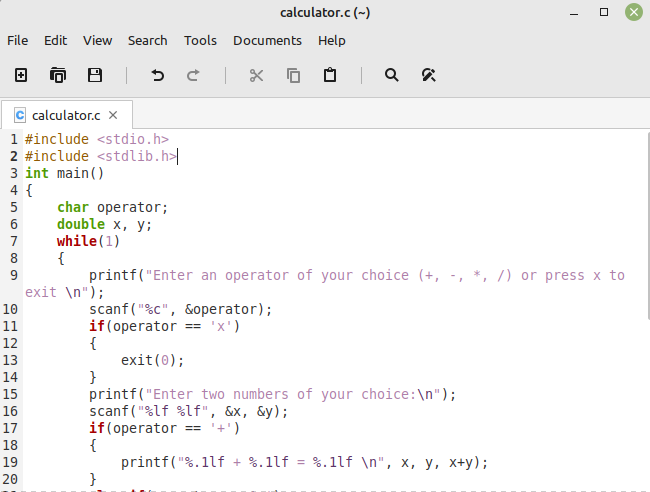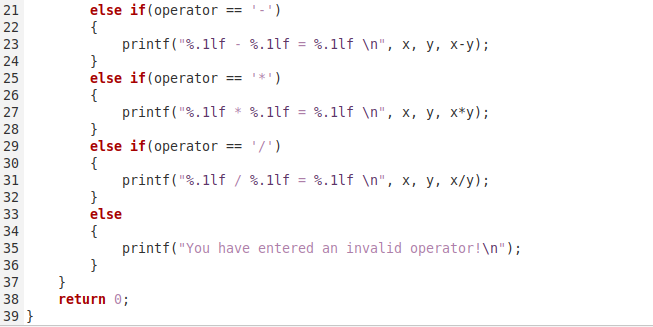In this program, we have declared an operator variable to take the operator as input from the user. Then, we have asked the user to enter two numbers of his choice. After that, we have used multiple “if-else” statements to check the operator entered by the user, and depending upon that, we have performed the specified operation on the entered numbers. After that, we also have an additional “else” statement to cater to the erroneous inputs. Moreover, we have enclosed this whole logic inside a “while” loop to make this program iterative. This program will keep asking the user to perform further calculations until they presses the “x” key to exit the program.

This program is compiled using the command shown below:

\$ gcc calculator.c –o calculator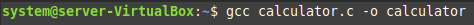Then, this program is executed by using the following command:

\$ ./calculator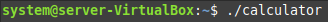Upon execution, we were asked to enter an operator of our choice. We entered the addition operator as shown in the image below: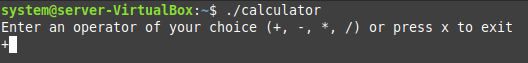Then, we were asked to enter the two numbers of our choice. We entered “22” and “27” as shown in the following image: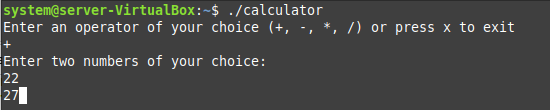The output of this operation is shown in the image below. You can also notice that this program did not terminate after displaying this output. Rather, we were asked to enter another operator of our choice which means that we have successfully managed to create an interactive calculator program in the C programming language using the “if-else” statement.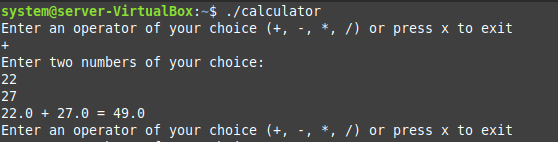Method # 2: Using the switch-case Statement

Now, we will create the same calculator program in C with the “switch-case” statement. For that, the C program is as follows: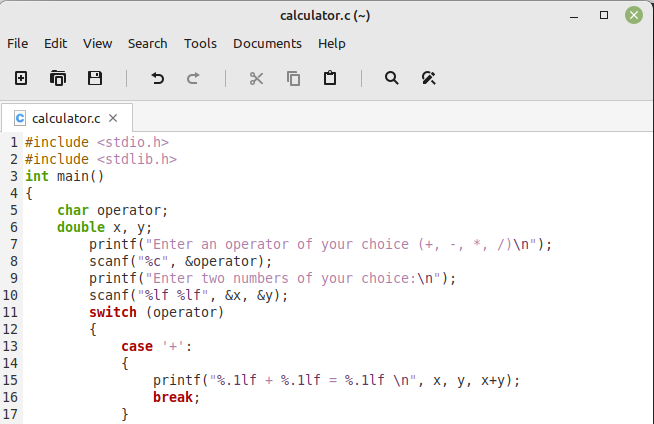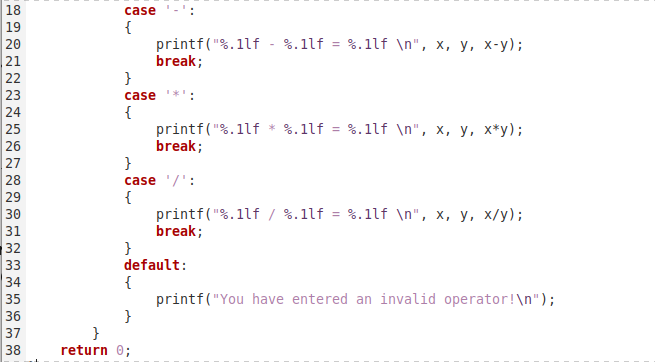In this program, we have again asked the user to enter an operator as well as two numbers of his choice. Then, we have used a “switch” statement on the “operator” variable and defined different cases to perform the desired operation depending upon the entered operator. Finally, there is a default case to cater to the erroneous input.

When we executed this script, we were asked to enter an operator of our choice. This time we entered the subtraction operator as shown in the image below: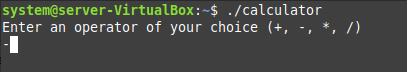Then, we were asked to enter two numbers on which the subtraction operation will be performed. These two numbers are shown in the following image: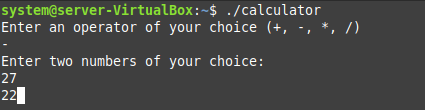The result of our subtraction operation is shown in the image below: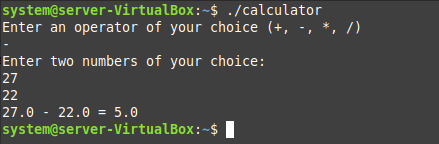## Conclusion

This article discussed the two different methods of writing a calculator program in the C programming language. The first method was based on the usage of the “if-else” statement whereas the second method made use of the “switch-case” statement. Now, you can follow any method of your choice to create your very own calculator in the C programming language.

Source: linuxhint.com